## Fluids

•sgbaek
Subscriber

Dear Experts

This is my first time to use the ANSYS Fluent package, and I hope I can get some help from you.

Here is my background: I am trying to model a transient pipe flow into vacuum thru a nozzle as shown below. (transient, 2d planar, density based, k-epsilon model, energy equation with ON).  The working gas is set to be helium with "ideal gas" For this kind of model (compressible flow), the boundary conditions used are the pressure inlet and outlet conditions, as several other tutorials have shown(  e.g., a YouTube tutorial:

) So as shown below, I have one pressure inlet boundary condition at the inlet (1000 torr, 300 K) and the pressure outlet boundary conditions (0 pressure assigned) around the simulation  domain. The operating condition is set to 0 to mock up a vacuum (I am aware that in this vacuum condition, FLUENT might not be doing the best).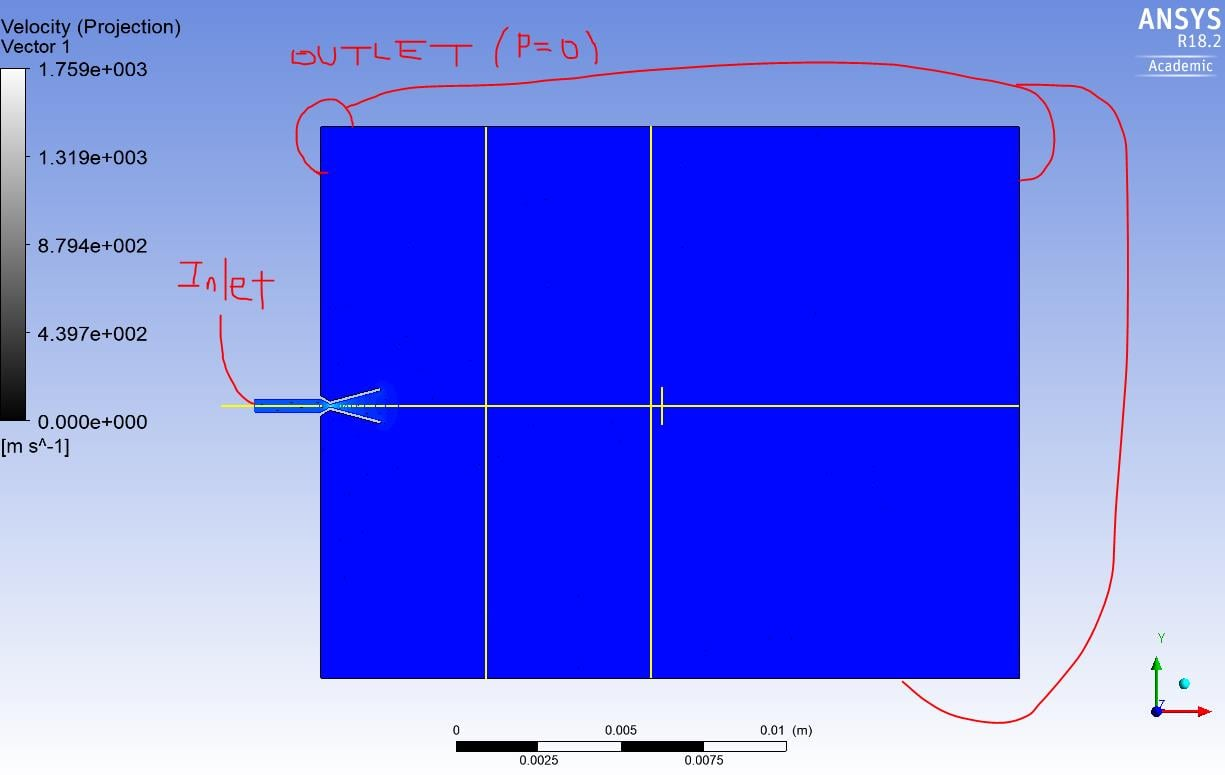Here is my problem: as I am trying to model a propagation of a gas along the pipe (it is a short pipe currently with a length of 2mm), I am interested in transient situation in order to compare the pipe flow evolution along the pipe and in the vacuum chamber. My expectation was that at the zeroth step in the transient calculation,  there is a step function like pressure drop (as indicated by light blue) because there is no gas down the pipe except at the inlet location.  However, ANSYS fluent result show that, even at t=0, the pressure profile shows a linear pressure decrease along the tube (shown below), and there is a finite density and velocity along the tube (not shown).  So clearly, Fluent have propagated the flow down the pipe already at the t=0 step, which is not realistic.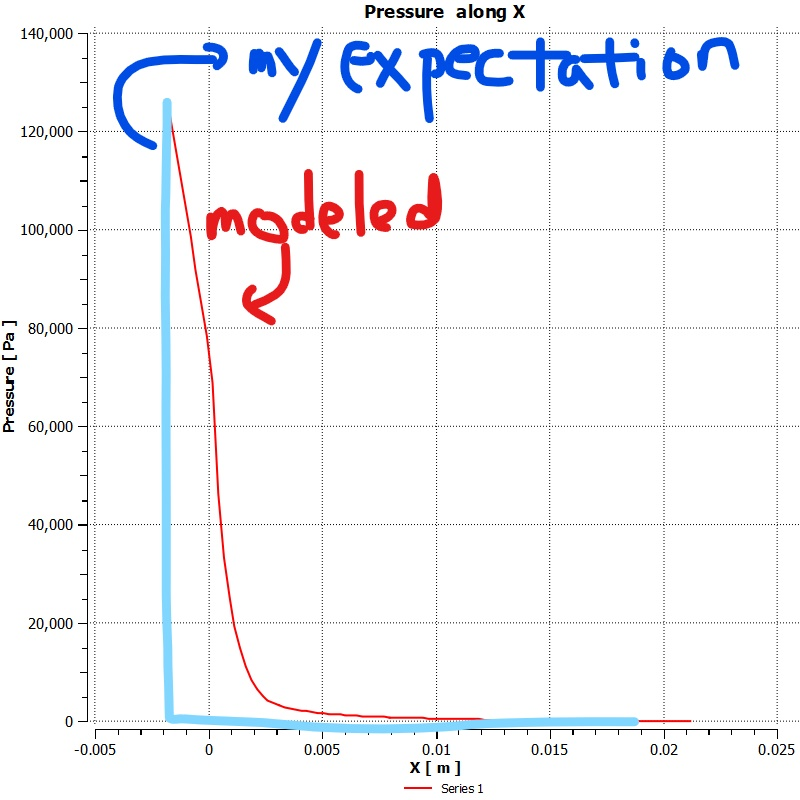I do not think that I correctly modeled the situation. I have tried different boundary conditions (such as mass flow inlet), but the solver did not like it.

Is there another approach (or boundary conditions) to properly model this kind of compressible flow propagation?

John

•Raef.Kobeissi
Subscriber

Hi,

what do you mean that at time =0 Fluent has propagated the flow? the result from the first time step is not showing t=0 it would be showing the first time step based on what you've assigned it.

Cheers

•sgbaek
Subscriber

Dear Raef

By t=0, I meant the step 0 in the output (e.g., Time =0 ), as shown below in the first figure. (At x=0 is the choke point.)  Should I understand "Step 0 " as the result of the first iteration, or the result of the initialization internally done in ANSYS? FYI, I used the "hybrid" initialization. If the latter,  I think that it is not a good initialization based on my reasoning as following: since my time step is 1e-7 second, I was thinking that this pressure and velocity profile (shown below) are not physically possible because there needs a certain amount of time for flow to propagate starting from the inlet point.

For example, the another figure below shows the velocity profile at Step 0 with  the velocity of 100 m/sec. For the given time step of 1e-7 sec, I thought that the flow should only have propagated 1e-5 m (=100 m/sec * 1e-7 sec), whereas the pressure and velocity profile along the x direction (2 cm in my simulation domain) are already formed. (Please note that the peak in the velocity profile is due to some kind of transient event, which may not be a real phenomenon -in the last figure, I am attaching a 2D velocity profile at Step 76 (the bottom most figure) which shows the structure apart from the one I am interested in. The structure separated from the nozzle propagates away and never appears again if sufficient amount of time is advanced. )

In summary, I am confused because of this evolution of fluid velocity (similarly, density) profile in the region where I think there should be no fluid at the given time step (say Step 0, 1,2) because the flow just did not have enough time to have propagated those regions. I guess that maybe I am not fully aware of how FEM works, and I appreciate your comment/guidance on this matter.

Thanks,

John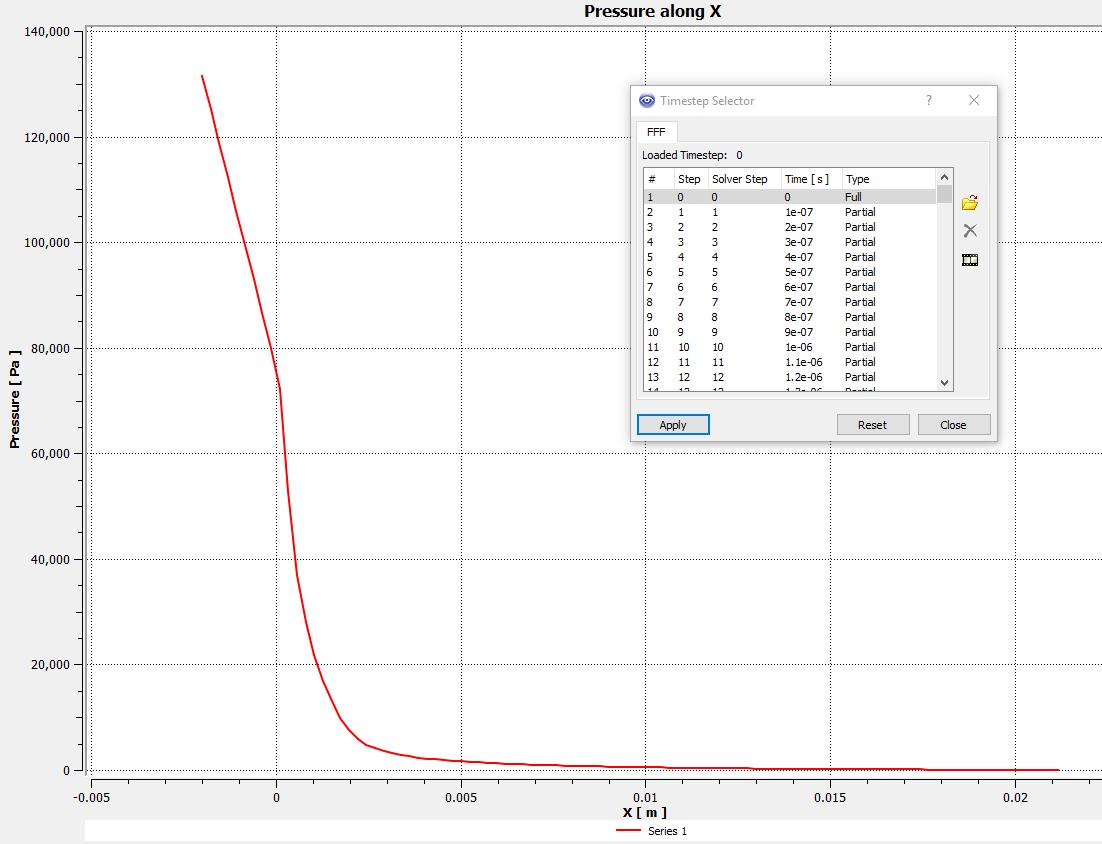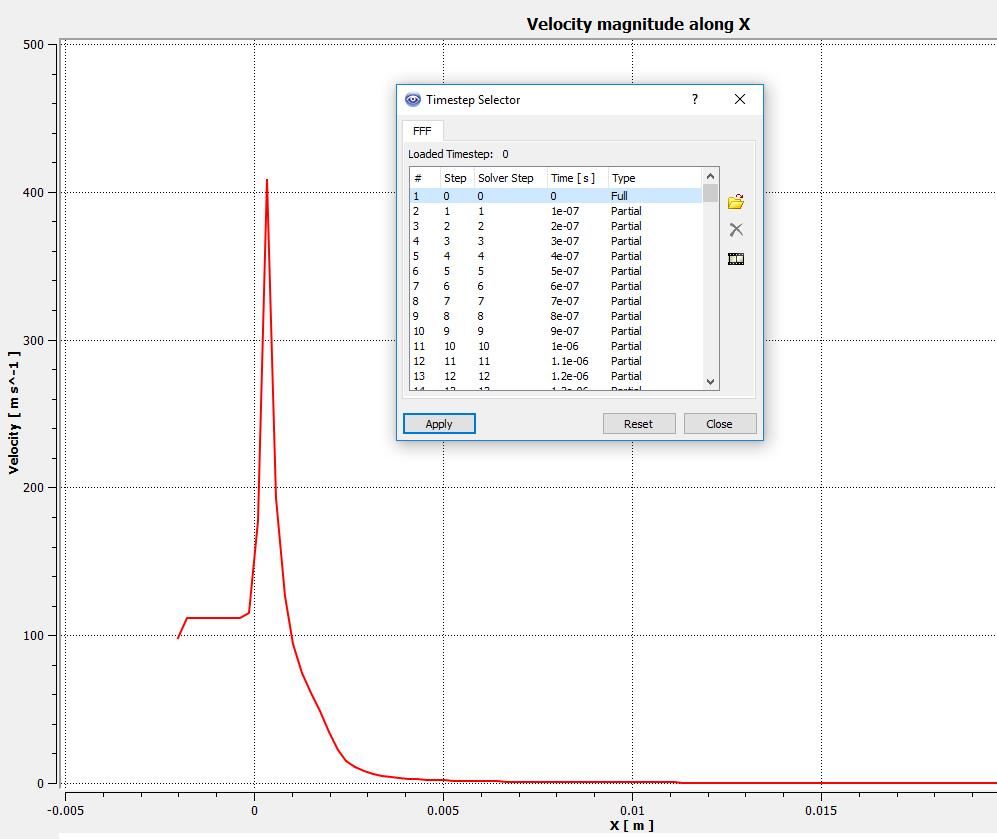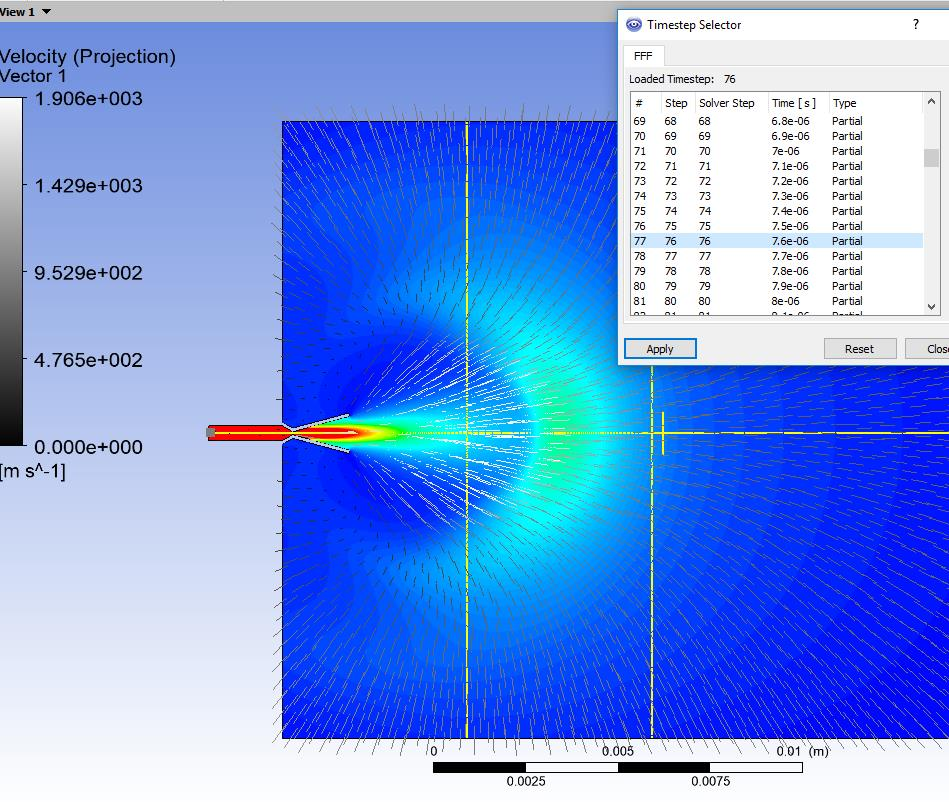•Raef.Kobeissi
Subscriber

Hello,

when you initialise a field you give it an initial value for its velocity and pressure nodes/cells all over the domain at t=0  (and other variables)

cheers

•sgbaek
Subscriber

Dear Raef

I appreciate your suggestion. Regarding to your suggestion, I have updated results and one follow-up question for you,which are not posted in "finite values inside the simulation domain with hybrid initialization at t =0'.

Thank you very much.

John

•tcs engineer
Subscriber

Dear sgbaek,

I am having a similar problem to create vacuum in ansys fluent,

problem is related to heat transfer in Al plates through the bolted joints,

one of the method i attempt creating to fluid zone and air with low properties and operating pressure 0. but i still not getting the correct results,

request you help me with this issue,

Regards,

Vijay

•DrAmine
Ansys Employee

Use Fluent and plot the pressure profile along the line you are using after initialization and share it with us